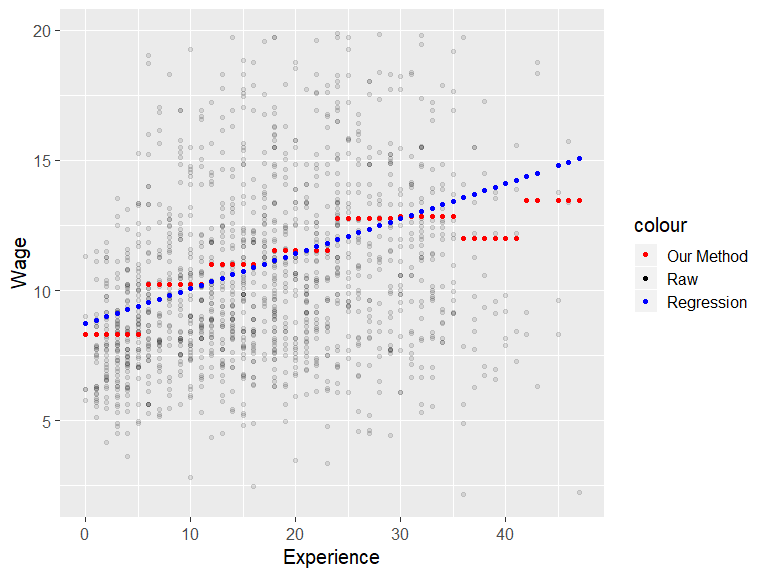# Lecture 27 Explaining Better - Regression

## Final Exam

• Combination of both programming and causal inference methods
• Everything is fair game
• There will also be a subjective question in which you take a causal question, develop a diagram, and perform the analysis with data I give you
• Slides and dagitty will be available, no other internet

## This Week

• We’ll be doing a little bit of review this last week
• And also talking about other ways of explaining data beyond what we’ve done
• This last week of material will not be on the final but it will be great prep for any upcoming class you take on this, or if you want to apply the ideas you’ve learned in class in the real world

## Explaining Better

• So far, all of our methods have had to do with explaining one variable with another
• After all, causal inference is all about looking at the effect of one variable on another
• If that explanation is causally identified, we’re good to go
• Or, if that explanation is on a back door of what we’re interested in, we’ll explain what we can and take it out

## Explaining Better

• The way that we’ve been explaining `A` with `B` so far:
• Take the different values of `B` (if it’s continuous, use bins with `cut()`)
• For observations with each of those different values, take the mean of `A`
• That mean is the “explained” part, the rest is the “residual”

## Explaining Better

• Now, this is the basic idea of explaining - what value of `A` can we expect, given the value of `B` we’re looking at?
• But this isn’t the only way to put that idea into action!

## Regression

• The way that explaining is done most of the time is with a method called regression
• You might be familiar with regression if you’ve taken ISDS 361A
• But here we’re going to go more into detail on what it actually is and how it relates to causal inference

## Regression

• The idea of regression is the same as our approach to explaining - for different values of `B`, predict `A`
• But what’s different? In regression, you impose a little more structure on that prediction
• Specifically, when `B` is continuous, you require that the relationship between `B` and `A` follows a straight line

## Regression

• Let’s look at wages and experience in Belgium
``````library(Ecdat)
data(Bwages)

#Explain wages with our normal method
Bwages <- Bwages %>% group_by(cut(exper,breaks=8)) %>%
mutate(wage.explained = mean(wage)) %>% ungroup()

#Explain wages with regression
#lm(wage~exper) regresses wage on exper, and predict() gets the explained values
Bwages <- Bwages %>%
mutate(wage.reg.explained = predict(lm(wage~exper)))

#What's in a regression? An intercept and a slope! Like I said, it's a line.
lm(wage~exper,data=Bwages)``````
``````##
## Call:
## lm(formula = wage ~ exper, data = Bwages)
##
## Coefficients:
## (Intercept)        exper
##      8.7349       0.1345``````

## Regression## Regression

• Okay, so it’s the same thing but it’s a straight line. Who cares?
• Regression brings us some benefits, but also has some costs (there’s always a tradeoff…)

## Regression Benefits

• It boils down the relationship to be much simpler to explain
• Instead of reporting eight different means, I can just give an intercept and a slope!
``````##
## Call:
## lm(formula = wage ~ exper, data = Bwages)
##
## Coefficients:
## (Intercept)        exper
##      8.7349       0.1345``````
• We can interpret this easily as “one more year of `exper` is associated with 0.134501 higher wages”

## Regression Benefits

• This makes it much easier to explain using multiple variables at once
• This is important when we’re doing causal inference. If we want to close multiple back doors, we have to control for multiple variables at once. This is unwieldy with our approach
• With regression we just add another dimension to our line and add another slope! As many as we want
``lm(wage~exper+educ+male,data=Bwages)``
``````##
## Call:
## lm(formula = wage ~ exper + educ + male, data = Bwages)
##
## Coefficients:
## (Intercept)        exper         educ     maleTRUE
##      1.0375       0.2006       1.9290       0.0767``````

## Regression Benefits

• The fact that we’re using a line means that we can use much more of the data
• This increases our statistical power, and also reduces overfitting (remember that?)
• For example, with regression discontinuity, we’ve been only looking just to the left and right of the cutoff
• But this doesn’t take into account the information we have about the trend of the variable leading up to the cutoff. Doing regression discontinuity with regression can!

## Regression Benefits

• Take this example from regression discontinuity